# Quantum Circuit Proof Notes

I'm going through some lecture notes on quantum computing by Childs. The lecture notes are dense, so I'm rewriting the formulas and adding personal notes to help me understand. On a quantum computer, any unitary operation can be applied to qubits, in theory. This section deals with approximating arbitrary unitary gates.

## Preliminaries

A unitary gate corresponds to a unitary matrix: any matrix that meets the following condition.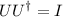The adjoint of a unitary gate is its own inverse.

Conveniently, the determinent of a unitary matrix is always.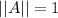In the context of quantum computing, this is easy to remember. The application of any quantum circuit starts and ends with a normalized ket,. The ket must be normalized, a unit vector, for the sum of all outcome probablities to equal, unity. Knowing this, it's easy to remember that any quantum circuit must take a normalized input ket to a normalized output ket. A matrix with a determenent ofis guranteed to preserve the magnitude of its input vector. This is a bit confusing; I've mixed around cause and effect, but it does make it easy to remember that unitary gates have a determenent of.

## Subadditivity of Errors

On an actual quantum computer, actual gate operations are only approximations of ideal gate operations. Unitarity is guranteed, but there will be some error. We can define the error as the determinent of the difference between some desired gate matrix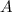and an actual gate matrix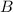: that is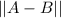. If we have a threshold, then our approximation is fine so long as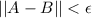.

It is of great interest how the error rate of multiple operations grow. We will prove that the error rate of a sequence of gates within the error threshold is at most the sum of each gate's error rate: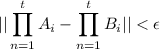The proof is by induction.

In the base case,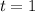. This is trivial.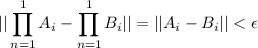The recursive case: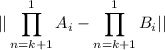We can add in these terms because they add to zero.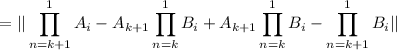By the triangle inequality, we know that the sum of the magnitudes is less than or equal to the magnitude of the sum; the sum of a triangles two shortest sides can only be as long as the longest side.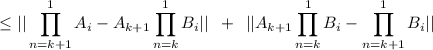We can factor some terms.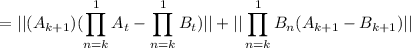Now remember that A and B are both guranteed to be unitary. They both have a deteriment of 1. Fortunately, the determinent of products is the product of determinents: || AB || = || A || || B ||.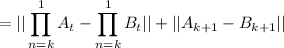So we have our recursive case: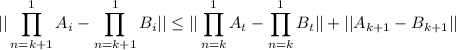This shows a linear error growth.

## Examples

To drive this home, here's a concrete example with a three gate system.

Suppose we have an ideal program: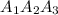And an actual program: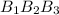We assume each gate is within some known error bound.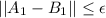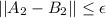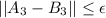Now lets expand our program's error rate and see what we get: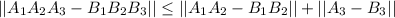Let's expand the 2 gate program: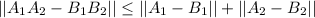If we combine the two equations, we see that the error rate of our entire program is less than or equal to the sum of the individual gate error rates.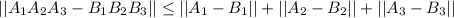This is what was expected. Awesome!

## Constructing Qubits

This is under construction.